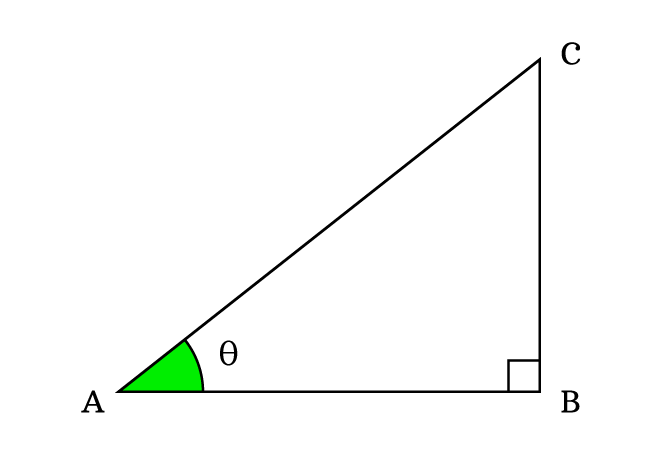# Quotient identities

## Formulas

$(1).\,\,\,\,\,\,$ $\dfrac{\sin{\theta}}{\cos{\theta}} \,=\, \tan{\theta}$

$(2).\,\,\,\,\,\,$ $\dfrac{\cos{\theta}}{\sin{\theta}} \,=\, \cot{\theta}$

A mathematical relation of two trigonometric functions in quotient form with another trigonometric function is called the quotient trigonometric identity.

## Introduction

A trigonometric function is appeared with another trigonometric function in division form in some cases but it is not always possible to divide a trigonometric function by another trigonometric function. However, there are two possible cases in which the quotient of two trigonometric functions is also a trigonometric function.

The two possible cases are used as formulas in trigonometry. They are called the quotient trigonometric identities and simply called as quotient identities.When the angle of a right triangle is represented by theta. The sine, cosine, tangent and cotangent functions are written as $\sin{\theta}$, $\cos{\theta}$, $\tan{\theta}$ and $\cot{\theta}$ respectively.

### Sine by Cosine Identity

The quotient of sine by cosine at an angle is equal to the tangent at that angle.

$\dfrac{\sin{\theta}}{\cos{\theta}} \,=\, \tan{\theta}$

### Cosine by Sine Identity

The quotient of cosine by sine at an angle is equal to the cotangent of that angle.

$\dfrac{\cos{\theta}}{\sin{\theta}} \,=\, \cot{\theta}$

Latest Math Topics
Jun 26, 2023
Jun 23, 2023

Latest Math Problems
Jul 01, 2023
Jun 25, 2023
###### Math Questions

The math problems with solutions to learn how to solve a problem.

Learn solutions

Practice now

###### Math Videos

The math videos tutorials with visual graphics to learn every concept.

Watch now

###### Subscribe us

Get the latest math updates from the Math Doubts by subscribing us.